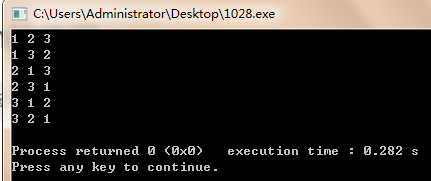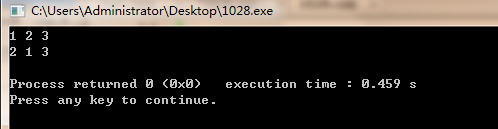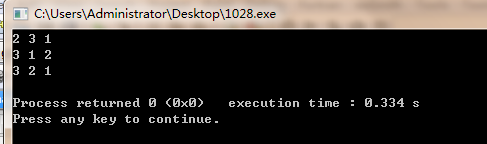# 关于对c++中的STL中全排列函数next_permutation的使用

1.next_permutation

``组合数学中经常用到排列，这里介绍一个计算序列全排列的函数：next_permutation（start,end），和prev_permutation（start,end）。这两个函数作用是一样的，区别就在于前者求的是当前排列的下一个排列，后一个求的是当前排列的上一个排列。至于这里的“前一个”和“后一个”，我们可以把它理解为序列的字典序的前后，严格来讲，就是对于当前序列pn，他的下一个序列pn+1满足：不存在另外的序列pm，使pn<pm<pn+1.``

2.对于next_permutation函数，其函数原型为：

``````  #include <algorithm>

bool next_permutation(iterator start,iterator end)``````

3.我们来看下面这个例子：

``````#include <iostream>
#include <algorithm>
using namespace std;
int main()
{
int num={1,2,3};
do
{
cout<<num<<" "<<num<<" "<<num<<endl;
}while(next_permutation(num,num+3));
return 0;
}
``````

4.输出结果为（排序完成的标志是所有数实现倒序排列）：5.当我们把while(next_permutation(num,num+3))中的3改为2时，输出就变为了(排序完成的标志是前两个数实现倒序排列)：6.由此可以看出，next_permutation(num,num+n)函数是对数组num中的前n个元素进行全排列，同时并改变num数组的值。----------------------------------------------------------------------------------------------------------------------------------

``````#include <stdio.h>
#include <algorithm>
using namespace std;
int main(){
int n;
while(scanf("%d",&n)&&n){//这句话排除了n等于0的情况
int a;
for(int i=0;i<n;i++){
scanf("%d",&a[i]);
}
sort(a,a+n);//可以自行测试一下删除后的结果
do{
for(int i=0;i<n;i++)
printf("%d ",a[i]);
printf("\n");
}while(next_permutation(a,a+n));
}
return 0;
}``````

3

1 0 2

0 1 2
0 2 1
1 0 2
1 2 0
2 0 1
2 1 0

1 0 2
1 2 0
2 0 1
2 1 0

prev_permutation（a,a+n）是相对于前者为逆序的。

THE END